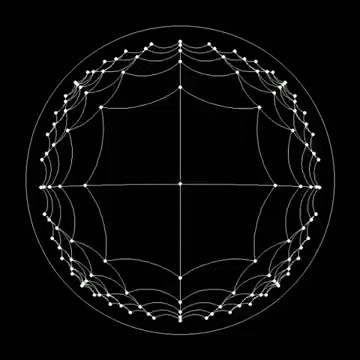top of page• Floney Yang

# Primitive Alterations and Hyperbolic Space

Updated: Apr 19, 2021

Collision detection for 4D objects

This week I have attempted several methods to generate collision detection for 4D objects. The general idea is to send the player’s transform position to the raymarch shader and use the distance function in the raymarch shader to detect distance. Currently, I am still trying to figure out how to communicate this data through C# scripts and shader. In my next step, I will attempt to figure out how to use a ray marcher to check the distance to a given transform, and if the distance is smaller than zero, the transform moves in a different direction.

While encountering blockers in collision detection simulation, I used the rest of my time researching other possible higher dimensional/raymarching topics.

Primitive Alterations

Elongating is a useful way to construct new shapes. It basically splits a primitive in two (four or eight), moves the pieces apart and connects them.```float opElongate( in sdf3d primitive, in vec3 p, in vec3 h )
{
vec3 q = p - clamp( p, -h, h );
return primitive( q );
}

float opElongate( in sdf3d primitive, in vec3 p, in vec3 h )
{
vec3 q = abs(p)-h;
return primitive( max(q,0.0) ) + min(max(q.x,max(q.y,q.z)),0.0);
}```

Primitive Combinations

Union, Subtraction, Intersection

These are the most basic combinations of pairs of primitives you can do. They correspond to the basic boolean operations.

```float opUnion( float d1, float d2 ) {  min(d1,d2); }
float opSubtraction( float d1, float d2 ) { return max(-d1,d2); }
float opIntersection( float d1, float d2 ) { return max(d1,d2); }```

Fractals

Fractals are simple infinitely repeated patterns that are self similar across different scales.The Mandelbulb

This 3D Mandelbulb can be achieved by squaring the 2D Mandelbrot equation: zn+1=z2n+c

But first, let us briefly return to the 2D Mandelbrot equation: zn+1= zn2+c

Building Hyperbolic Space in Unity1. Technique: Tile Mapping

• Change different square per vertex to use different levels

• Represent tile coordinates as string of steps away from the origin

• Recursively expand from origin, if a tile already exists, skip and move on

2. Represent location, rotation, coordinates system

• In Unity 3D, this has been handled using a transformation matrix with all the information for position/rotation/movement

• Repeating the same concept for hyperbolic space, this time we use gyrovector to do matrix computation.

Objectives for next week

1. I will continue working on collision detection for 4D objects. I will create a class to store all information for a 4D objects and using a C# script to collect and compute data before sending data to a shader. In this way, the script that manages the transform of the player can better communicate with the distance function for ray marching.

2. I will implement more functions for primitive alterations to see what interesting new shapes can be generated through this method.

3. I will start implementing a 2D Fractal algorithm in Unity to see a possible visualization of fractals.

Learning Resources

1. Ray Marching/Distance functions: https://iquilezles.org/www/articles/distfunctions/distfunctions.htm

Reference

3. Gif used: https://imgur.com/V96Zy7b

79 views

See All
bottom of page